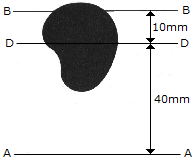# Engineering Mechanics - Moments of Inertia - Discussion

### Discussion :: Moments of Inertia - General Questions (Q.No.4)

4.The irregular area has a moment of inertia about the AA axis of 35 (106) mm4. If the total area is 12.0(103) mm2, determine the moment of inertia if the area about the BB axis. The DD axis passes through the centroid C of the area.

 [A]. IBB = 5.00(106 ) mm4 [B]. IBB = 17.00(106 ) mm4 [C]. IBB = 16.80(106 ) mm4 [D]. IBB = 55.4(106 ) mm4

Explanation:

No answer description available for this question.

 Sujin said: (Mar 26, 2012) Ibb=Idd+A(r*r) Iaa=Idd+A(x*x) r=10mm,x=40mm Ibb-Iaa=A(r*r-x*x) Ibb=Iaa+A(r*r-x*x) Ibb=[35+12(100-1600)]*100000 Ibb=17*10^6mm^4

 Aniruddha said: (Jun 5, 2012) First find Idd ?. Idd = Iaa-(area*Distance betn DD and AA^sq.) = Iaa-(Area*40^2) = (35X10^6) - ((12X10^3)X40^2) = 15.8 x 10^6 Now find Ibb = Idd + Area X h^2 = (15.8x10^6) + (12x10^3)(10^2) ANS = 17 X 10^6

 Gowthamy said: (Aug 21, 2013) Ibb = Idd+12*10^3*100. = (35*10^6)-(12*10^3*1600)+12*10^3*100.

 Rupesh said: (Aug 14, 2017) Ibb = Idd+12*10^3*100. = (35*10^6)-(12*10^3*1600)+12*10^3*100.